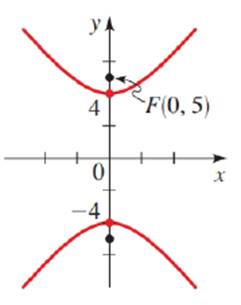Chapter 12.CR, Problem 39E### Algebra and Trigonometry (MindTap ...

4th Edition
James Stewart + 2 others
ISBN: 9781305071742

#### Solutions

Chapter
Section### Algebra and Trigonometry (MindTap ...

4th Edition
James Stewart + 2 others
ISBN: 9781305071742
Textbook Problem

# Finding the Equation of a Conic Find an equation for the conic whose graph is shown.To determine

To find:

An equation of the given conic.

Explanation

Given:

The graph of the conic:

Figure (1)

Approach:

The equation of vertical hyperbola is y2a2x2b2=1 with foci (0,±c), vertices (0,±a) and b2=c2a2.

Calculation:

From figure (1), focus is F(0,5) and vertices are (0,±4).

Therefore, c=5 and a=4

### Still sussing out bartleby?

Check out a sample textbook solution.

See a sample solution

#### The Solution to Your Study Problems

Bartleby provides explanations to thousands of textbook problems written by our experts, many with advanced degrees!

Get Started